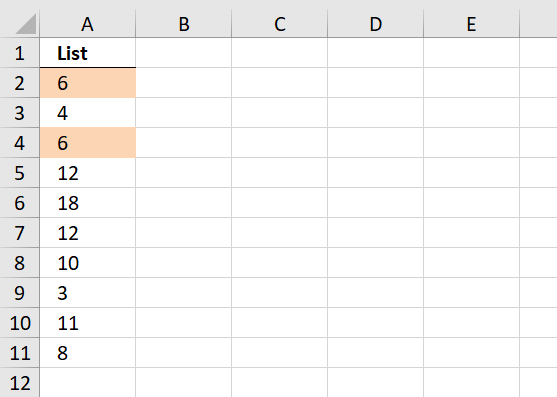Author: Oscar Cronquist Article last updated on November 22, 2018

Question: How do I highlight the smallest duplicate value in a column using conditional formatting?Conditional formatting formula in A2:

=MIN(IF(COUNTIF(\$A\$2:\$A\$11, \$A\$2:\$A\$11)>1, \$A\$2:\$A\$11, ""))=A2

How to apply conditional formatting

1. Select cell range
2. Go to "Home" tab
3. Press with left mouse button on Conditional formatting
4. Press with left mouse button on "New Rule.."
5. Press with left mouse button on "Use a formula to determine what cells to format"
6. Copy the above conditional formatting formula to "Format values where this formula is true:"
7. Press with left mouse button on Format button
8. Select a formatting you like.
9. Press with left mouse button on OK
10. Press with left mouse button on OK

### Explaining CF formula in cell A2

#### Step 1 - Identify duplicates

The COUNTIF function counts values based on a condition or criteria.

COUNTIF(\$A\$2:\$A\$11, \$A\$2:\$A\$11)>1

becomes

COUNTIF({6;4;6;12;18;12;10;3;11;8}, {6;4;6;12;18;12;10;3;11;8})>1

becomes

{2;1;2;2;1;2;1;1;1;1}>1

and returns

{TRUE; FALSE; TRUE; TRUE; FALSE; TRUE; FALSE; FALSE; FALSE; FALSE}.

#### Step 2 - Extract duplicate numbers

The IF function uses a logical expression in order to determine which argument to return.

IF(COUNTIF(\$A\$2:\$A\$11, \$A\$2:\$A\$11)>1, \$A\$2:\$A\$11, "")

becomes

IF({TRUE; FALSE; TRUE; TRUE; FALSE; TRUE; FALSE; FALSE; FALSE; FALSE}, \$A\$2:\$A\$11, "")

becomes

IF({TRUE; FALSE; TRUE; TRUE; FALSE; TRUE; FALSE; FALSE; FALSE; FALSE}, {6;4;6;12;18;12;10;3;11;8}, "")

and returns

{6;"";6;12;"";12;"";"";"";""}.

#### Step 3 - Identify the smallest number in array

The MIN function extracts the minimum value in a cell range or array.

MIN(IF(COUNTIF(\$A\$2:\$A\$11, \$A\$2:\$A\$11)>1, \$A\$2:\$A\$11, ""))

becomes

MIN({6;"";6;12;"";12;"";"";"";""})

and returns 6.

#### Step 4 - Compare smallest number to current cell

MIN(IF(COUNTIF(\$A\$2:\$A\$11, \$A\$2:\$A\$11)>1, \$A\$2:\$A\$11, ""))=A2

becomes

6=A2

becomes

6=6

and returns TRUE. Cell A2 is highlighted.

### Get Excel *.xls file

highlight-smallest-duplicate-value-in-a-column.xls